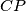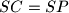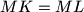### IMO Shortlist 2010 problem G2

Kvaliteta:
Avg: 2.5
Težina:
Avg: 6.0
Let$P$ be a point interior to triangle$ABC$ (with$CA \neq CB$). The lines$AP$,$BP$ and$CP$ meet again its circumcircle$\Gamma$ at$K$,$L$, respectively$M$. The tangent line at$C$ to$\Gamma$ meets the line$AB$ at$S$. Show that from$SC = SP$ follows$MK = ML$.

Proposed by Marcin E. Kuczma, Poland
Source: Međunarodna matematička olimpijada, shortlist 2010